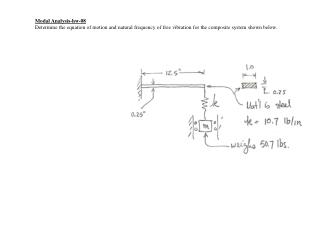DownloadDownload PresentationModal Analysis-hw-08 Determine the equation of motion and natural frequency of free vibration for the composite system s

# Modal Analysis-hw-08 Determine the equation of motion and natural frequency of free vibration for the composite system s

Télécharger la présentation## Modal Analysis-hw-08 Determine the equation of motion and natural frequency of free vibration for the composite system s

- - - - - - - - - - - - - - - - - - - - - - - - - - - E N D - - - - - - - - - - - - - - - - - - - - - - - - - - -
##### Presentation Transcript

1. Modal Analysis-hw-08 Determine the equation of motion and natural frequency of free vibration for the composite system shown below.

2. Modal Analysis-hw-08 (cont’d)

3. Modal Analysis-hw-09 Determine the equation of motion and natural frequency of free vibration for the bell crank system shown below by ignoring the mass of the bell crank itself.

4. Modal Analysis-hw-09 (cont’d)

5. Modal Analysis-hw-10 Determine the equation of motion, natural frequency of free vibration, and normal modes for the double pendulum system shown below.

6. Modal Analysis-hw-10 (cont’d)

7. Modal Analysis-hw-11 Determine the equation of motion and natural frequency of free vibration for the composite system shown below using both the Newtonian and Lagrange equation methods. Assume the cables are inextensible.

8. Modal Analysis-hw-11 (cont’d)